# C220 – API Compressibility and Cpl

## Description

This calculates the volume flow rate adjusted to take pressure and compressibility into account.

Measurement of Petroleum Measurement Standards Chapter 11.2.1 – Compressibility Factors for Hydrocarbons: 0-90° API Gravity Range (1984)

Measurement of Petroleum Measurement Standards Chapter 11.2.1M – Compressibility Factors for Hydrocarbons: 638-1074 Kilograms per Cubic Metre Range (First Edition August 1984)

Measurement of Petroleum Measurement Standards Chapter 11.2.2 – Compressibility Factors for Hydrocarbons: 0.350-0.637 Relative Density (60°F/60°F) and -50°F to 140°F Metering Temperature (First Edition October 1986)

Measurement of Petroleum Measurement Standards Chapter 11.2.2M – Compressibility Factors for Hydrocarbons: 350-637 Kilograms per Cubic Metre Density (15°C) and -46°C to 60°C Metering Temperature (First Edition October 1986)

Kelton calculation reference C220

This calculation is used by the following calculations:

F028/K211 – API/Table 54 Density Referral

F023/K212 – API Density Referral

K229- GPA TP-25/27 – NGL and LPG Density Referral Calculation

F091 – GPA TP-25 – NGL and LPG Density Referral Calculation

F092 – GPA TP-27 – NGL and LPG Density Referral Calculation

F103 – API Table 24:1952 Density Referral

## Options

### Units of standard

• Metric

This option uses the metric versions of the API standards i.e. API 11.2.1M or API 11.2.2M

• US Customary

This option uses the versions of the API standards which use US customary units i.e. API 11.2.1 or API 11.2.2

### Density Range

• Auto

Automatically select the appropriate calculation

• 350 to 637 kg/m³

Calculate using Chapter 11.2.2M procedure

• 638 to 1074 kg/m³

Calculate using Chapter 11.2.1M procedure

### Vapour Pressure

Select this option to include the vapour pressure – the equilibrium bubble point pressure

### Rounding

The standard specifies the method of rounding used to generate the tables, You can either select to round as per the standard or use the full precision.

## Constants

If the metric option is selected, for API 11.2.1M the following constants are used to calculate compressibility factor

A = -1.62080 B = 2.1592 x 10-4 C = 0.79392 D = 2.326 x 10-3

If the US customary option is selected, for API 11.2.1 the following constants are used to calculate compressibility factor

A = -1.9947 B = 1.3427x 10-4 C = 0.87096 D = 4.2092 x 10-3

## Calculations

### For 350 to 637 kg/m3 (with rounding)

The correction for the pressure of the liquid is calculated by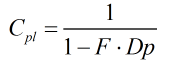Where the compressibility factor (F) is calculated by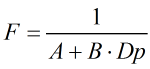Where DP = The pressure above the equilibrium bubble point pressure (Pe)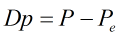The terms A and B are calculated as below

Note: the steps which are for the tabular display have been omitted and leaving only those pertaining to the calculation.

The temperature is converted to °C to use the same units as the standard.

Step 1: The temperature is converted to Rankine using the approximation described in the standard

Step 2: Convert density to g/cm3

Step 3: Obtain 3 significant figures

Step 4: If the metric option is selected convert the density (15°C) to density (60°F).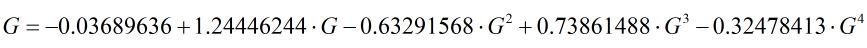Step 5: Convert the calculated density (60°F) in grams per cubic centimetre to relative density using the density of water at 60°F

Step 6: Obtain three significant decimal figures

Step 7: Calculate 96% of the critical temperature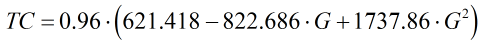Step 8: If the temperature in degrees Rankine TR is more than the near critical limit (TC). void the calculation, output a message, and start the next density and temperature set

Step 9: If the temperature in degrees Rankine TR is less than or equal to the near critical limit (TC). then calculate the A and B factors using steps 12 to 18.

Step 10: Calculate the A Factor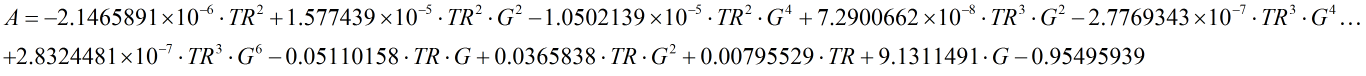Step 11: Convert the A Factor to kilopascals if metric is selected, to psi if US customary is selected.

Step 12: Round and scale the A factor to the nearest whole number

Step 13: Calculate the B Factor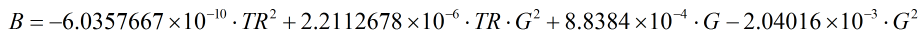Step 14: Round and scale the B factor to the nearest 0.001

### For 638 to 1074 kg/m3 (with rounding)

The basic equation forcalculating the compressibility factor (F) is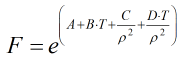Where A = Coefficient for compressibility factor B = Coefficient for compressibility factor C = Coefficient for compressibility factor D = Coefficient for compressibility factor T = Temperature in °C ρ = Density at 15°C

Back